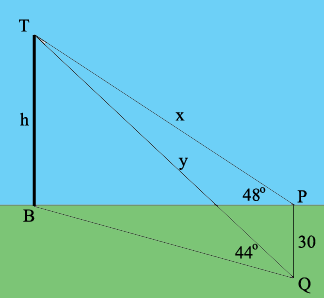SEARCH HOMEMath Central Quandaries & QueriesQuestion from ASIT, a student: A vertical aerial stands on a horizontal ground. A surveyor positioned due east of the aerial measures the elevation of the Top as 48 degree. He moves due south 30metre and measure the elevation as 44 degree. determine the height of the aerialHi,

In my diagram B is the bottom of the aerial, T is its top, P is the first surveyor position and Q is the second surveyor position. The distances are in metres.What trig function relates $x$ and $h$ to the measure of the angle $BPT?$

What trig function relates $y$ and $h$ to the measure of the angle $BQT?$

Now you have two equations but three unknowns, so you need another equation. Notice that angle $QPT$ is a right angle so triangle $QPT$ is a right triangle. Maybe Pythagoras can help.

PennyMath Central is supported by the University of Regina and The Pacific Institute for the Mathematical Sciences.